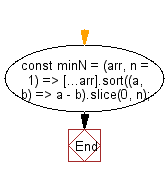# JavaScript: Get the n minimum elements from the provided array

## JavaScript fundamental (ES6 Syntax): Exercise-176 with Solution

Write a JavaScript program to get the n minimum elements from the provided array.
If n is greater than or equal to the provided array's length, return the original array (sorted in ascending order).

• Use Array.prototype.sort() combined with the spread operator (...) to create a shallow clone of the array and sort it in ascending order.
• Use Array.prototype.slice() to get the specified number of elements.
• Omit the second argument, n, to get a one-element array.
• If n is greater than or equal to the provided array's length, then return the original array (sorted in ascending order).

Sample Solution:

JavaScript Code:

``````//#Source https://bit.ly/2neWfJ2
const minN = (arr, n = 1) => [...arr].sort((a, b) => a - b).slice(0, n);
console.log(minN([1, 2, 3]));
console.log(minN([1, 2, 3], 2));
```
```

Sample Output:

```
[1,2]
```

Pictorial Presentation:Flowchart:Live Demo:

See the Pen javascript-basic-exercise-176-1 by w3resource (@w3resource) on CodePen.

Improve this sample solution and post your code through Disqus

What is the difficulty level of this exercise?

Test your Programming skills with w3resource's quiz.

﻿